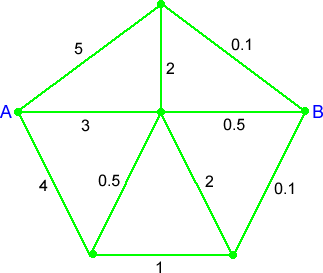You may also likeCubes

How many faces can you see when you arrange these three cubes in different ways?Pebbles

Place four pebbles on the sand in the form of a square. Keep adding as few pebbles as necessary to double the area. How many extra pebbles are added each time?Bracelets

Investigate the different shaped bracelets you could make from 18 different spherical beads. How do they compare if you use 24 beads?

Route Product

Route Product

There are lots of different routes from $A$ to $B$ in this diagram:The idea is to work out the product of the numbers on these different routes from $A$ to $B$. Let's say that in a route you are not allowed to visit a point more than once.

For example, we could have $3\times0.5$ but we couldn't have $3\times2\times5\times4\times1\times 0.1$ because that route passes through $A$ twice.

Which route or routes give the largest product?

Which route or routes give the smallest product?

Do you have any quick ways of working out the products each time?

[This problem is adapted from a SMILE Centre card.]

Why do this problem?

Multiplying decimals is perceived as a tricky task but this problem is written to encourage children to make the link between multiplying by a decimal and dividing by a whole number. This challenge also requires learners to work in a systematic way.

Possible approach

You might find it useful to leave pupils to have a go at this challenge without saying much at all by way of introduction. It would probably be helpful for them to have a paper copy of the grid.

After a few minutes invite them to comment on how they are going about the task. It would be useful to focus on two aspects. Firstly, the way that they are approaching the problem - how will they know they have looked at all the routes? This would be worth a discussion on having a system, such as starting with all the routes that begin by going up from A along the $5$ path, then those that start with the $3$ etc. Secondly, you could talk about their methods for calculating the products in each case. Some learners might suggest that multiplying by $2$ 'cancels out' multiplying by $0.5$, for example, and you can encourage them to explain why.

You could ask pupils to write each route (and its product) on a separate strip of paper which could then be stuck on the board in the plenary. Ask the class to organise the strips to help them decide whether any have been missed out. Answering the questions will then be very straightforward.

Key questions

How do you know you have looked at all the possible routes?
How are you calculating the product each time?

Possible extension

As an extension activity you could invite children to create their own grid using some criteria. For example, could they make the product of all routes $1$? (Perhaps without using the number $1$?) They could start by using the route design given and then make up their own route maps.

Possible support

It might help some learners to express the decimals as fractions before finding the products. This may well come up in the whole-class discussion.